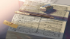Discussion about math, puzzles, games and fun.   Useful symbols: ÷ × ½ √ ∞ ≠ ≤ ≥ ≈ ⇒ ± ∈ Δ θ ∴ ∑ ∫  π  -¹ ² ³ °

You are not logged in.

## #1 2019-09-24 00:56:51

Hannibal lecter
MemberRegistered: 2016-02-11
Posts: 279

### exponent

Hi,

P = P0 * e^kt
where where P0 and initial quantities
e =  2.71828

We say that P and Q are growing or decaying at a continuous rate of k. (For example, k =0 .02 corresponds to a continuous rate of 2%.)

what is the meaning of continuous rate and when we say it's a constant rate ?

P = 5(1 .07)^s

in this other example the growth rate it 0.07 which is 7%

in the first example the growth rate was an exponent ! ( k value )

what changed ? how would the growth rate changing his places in a functions

Last edited by Hannibal lecter (2019-09-24 17:04:22)

Wisdom is a tree which grows in the heart and fruits on the tongue

Offline